Tamilnadu State Board New Syllabus Samacheer Kalvi 11th Maths Guide Pdf Chapter 7 Matrices and Determinants Ex 7.5 Text Book Back Questions and Answers, Notes.

## Tamilnadu Samacheer Kalvi 11th Maths Solutions Chapter 7 Matrices and Determinants Ex 7.5

Choose the correct or the most suitable answer from the given four alternatives.

Question 1.
If aij =  (3i – 2j) and A = [aij]3 × 2 is
(1)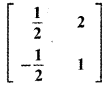(2)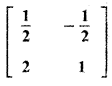(3)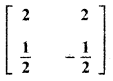(4)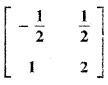(2)Explaination: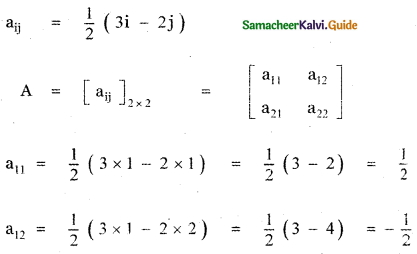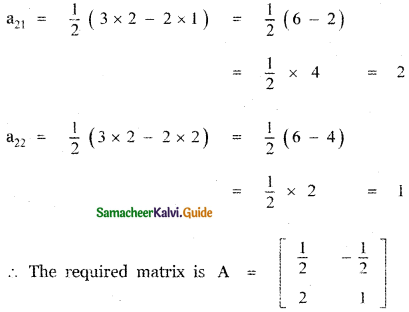Question 2.
What must be the matrix X, if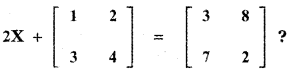(1)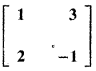(2)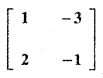(3)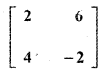(4)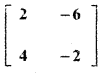(1)Explaination: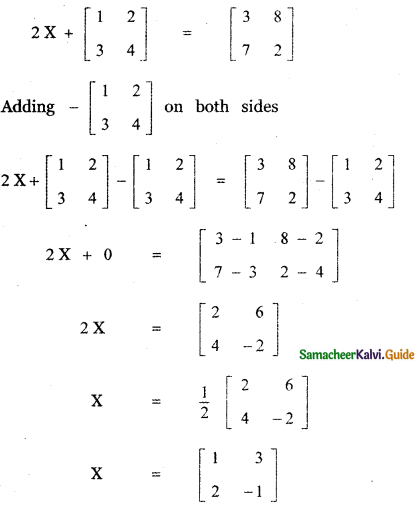Question 3.
Which one of the following is not true about the matrix $$\left[ \begin{matrix} 1 & 0 & 0 \\ 0 & 0 & 0 \\ 0 & 0 & 0 \end{matrix} \right]$$ ?.
(1) a scalar matrix
(2) a diagonal matrix
(3) an upper triangular matrix
(4) a lower triangular matrix
(1) a scalar matrix

Explaination:
Let A = $$\left[ \begin{matrix} 1 & 0 & 0 \\ 0 & 0 & 0 \\ 0 & 0 & 0 \end{matrix} \right]$$
(1) a scalar matrix – not true
(2) a diagonal matrix – true
(3) an upper triangular matrix – true
(4) a lower triangular matrix – true

[(1) A square matrix A = [aij]m × n is called a diagonal matrix if aij = 0 whenever i ≠ j
(2) A diagonal matrix whose entries along the principle diagonal are equal is called a scalar matrix.
(3) A square matrix is said to be an upper triangular matrix if all the elements below the main diagonal are zero.
(4) A square matrix is said to be a lower triangular matrix if all the elements above the main diagonal are zero.]Question 4.
If A and B are two matrices such that A + B and AB are both defined, then
(1) A and B are two matrices not necessarily of same order
(2) A and B are square matrices of same order
(3) Number of columns of A is equal to the number of rows of B
(4) A = B
(2) A and B are square matrices of same order

Explaination:
Given A and B are two matrices such that A + B and AB are both defined.
A + B defined means A and B are same order.
AB defined means , Number of columns of A equal to Number of rows of B.
A + B and AB are simultaneously defined.
Therefore A and B are of same order.

Question 5.
If A = $$\left[ \begin{matrix} λ & 1 \\ -1 & -λ \end{matrix} \right]$$, then for what value of λ, A2 = 0 ?
(1) 0
(2) ± 1
(3) – 1
(4) 1
(2) ± 1

Explaination: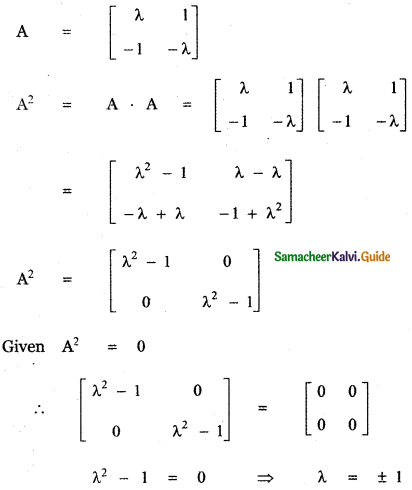Question 6.
If A = $$\left[ \begin{matrix} 1 & -1 \\ 2 & -1 \end{matrix} \right]$$, B = $$\left[ \begin{matrix} a & 1 \\ b & -1 \end{matrix} \right]$$ and (A + B)2 = A2 + B2, then the values of a and b are
(1) a = 4, b = 1
(2) a = 1, b = 4
(3) a = 0, b = 4
(4) a = 2, b = 4
(2) a = 1, b = 4

Explaination: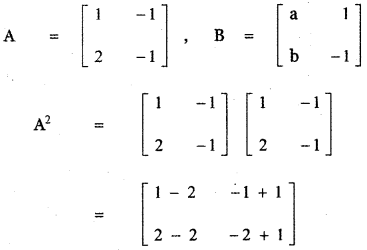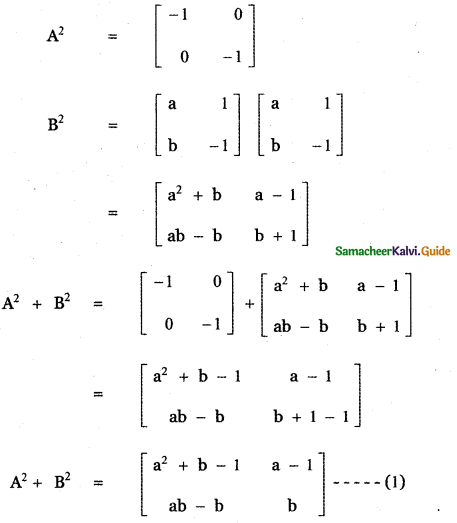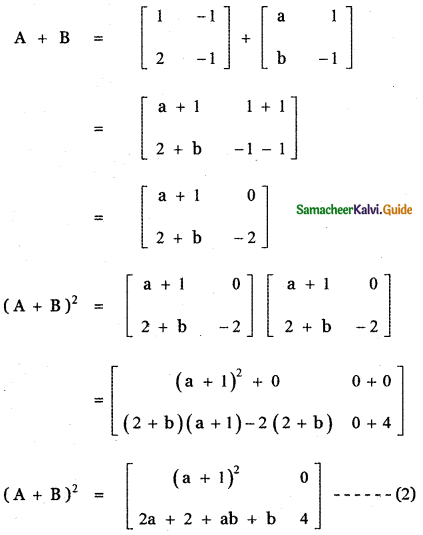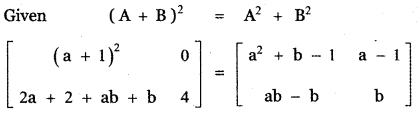L .2
Equating the like entrices
(a – 1)2 = a2 + b – 1 ………. (3)
o = a – 1 ………. (4)
2a+ 2 + ab + b = ab – b ………. (5)
4 = b ………. (6)
a = 1, b = 4Question 7.
If A = $$\left[ \begin{matrix} 1 & 2 & 2 \\ 2 & 1 & -2 \\ a & 2 & b \end{matrix} \right]$$
the equation AAT = 9I, where I is 3 × 3 identity matrix, then the ordered pair (a, b)
is equal to
(1) (2, -1)
(2) (- 2, 1)
(3) (2, 1)
(4) (- 2, – 1)
(4) (- 2, – 1)

Explaination: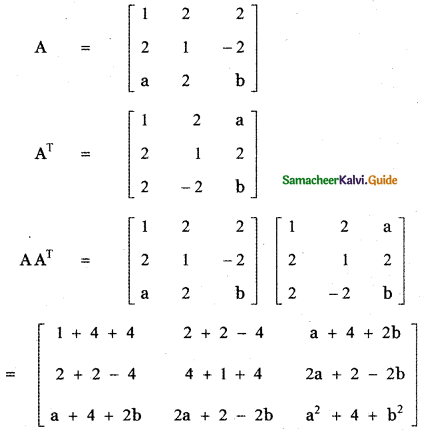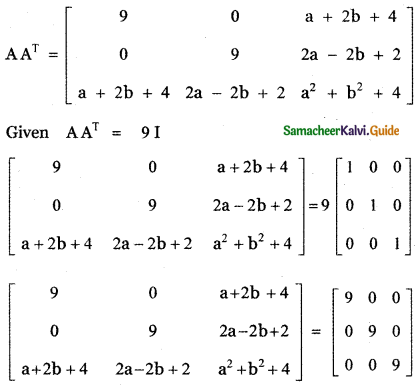Equating the like entries
a + 2b + 4 = 0 …………. (1)
2a – 2b + 2 = 0
a – b + 1 = 0 …………. (2)
a2 + b2 + 4 = 9 …………. (3)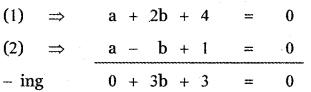3b = – 3 ⇒ b = -1
Substituting in equation (1) we get
a + 2 × – 1 + 4 = 0
a – 2 + 4 = 0
a = – 2
Substituting the values a = – 2 , b = – 1 in equation (3)
we have
(- 2)2 + (-1)2 + 4 = 9
4 + 1 + 4 = 9
9 = 9
∴ The required value of the ordered pair (a, b) is
(a, b) = (- 2, – 1)Question 8.
If A is a square matrix, then which of the following is not symmetric?
(1) A + AT
(2) AAT
(3) ATA
(4) AAT
(4) AAT

Explaination:
Given A is a square matrix.
A square matrix A is symmetric if AT = A

(1) A + AT
(A + AT)T = AT + (AT)T
= AT + A = A + AT
∴ A + AT is symmetric.

(2) AAT
(AAT)T = (AT)TAT
= AAT
∴ AAT is symmetric.

(3) ATA
(ATA)T = AT(AT)T
= ATA
∴ ATA is symmetric.

(4) A – AT
(A – AT)T = AT – (AT)T
= ATA
∴ A – AT is not symmetric.

Question 9.
If A and B are symmetric matrices of order n , where (A ≠ B) , then
(1) A + B is skew – symmetric
(2) A + B is symmetric
(3) A + B is a diagonal matrix
(4) A + B is a zero matrix
(2) A + B is symmetric

Explaination:
Given A and B are symmetric matrices of order n.
∴ AT = A and BT = B
A matrix A is skew symmetric if AT = – A
(1)(A + B)T = AT + BT = A + B
A + B is not skew symmetric.
(2)(A + B)T = AT+BT = A + B
∴ A + B is symmetric.
(3) A + B is a diagonal matrix is incorrect.
(4) A + B is a zero matrix is incorrect.Question 10.
If A = $$\left[ \begin{matrix} a & x \\ y & a \end{matrix} \right]$$ and if xy = 1, then det (AAT) is equal to
(1) (a – 1)2
(2) (a2 + 1)2
(3) a2 – 1
(4) (a2 – 1)2
(4) (a2 – 1)2

Explaination: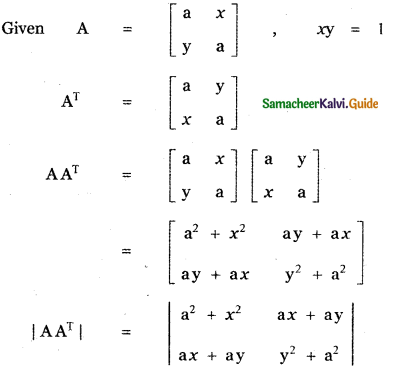= (a2 + x2) (y2 + a2) – (ax + ay) (ax + ay)
= a2 y2 + a4 + x2 y2 + a2 x2 – ((ax)2 + (ay)2 + 2 (ax)(ay))
= a2 x2 + a2 y2 + a4 + (xy)2 – a2 x2 – a2 y2 – 2a2 xy
= a4 + (1)2 – 2a2(1)
= a4 – 2a2 + 1
|AAT| = (a2 – 1)2

Question 11.
The value of x, for which the matrix A = $$\left[ \begin{matrix} { e }^{ x-2 } & { e }^{ 7+x } \\ { e }^{ 2+x } & { e }^{ 2x+3 } \end{matrix} \right]$$ is singular
(1) 9
(2) 8
(3) 7
(4) 6
(2) 8

Explaination: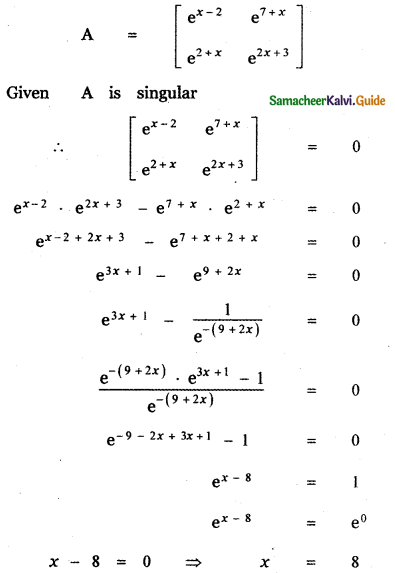Question 12.
If the points (x, – 2), (5, 2), (8, 8) are collinear , then x is equal to
(1) – 3
(2) $$\frac{1}{3}$$
(3) 1
(4) 3
(4) 3

Explaination:
Let the given points be (x1, y1) = (x, – 2) ,
(x2, y2) = (5, 2) and (x3, y3) = (8, 8)
The condition for the three points (x1, y1), (x2, y2) and (x3, y3) to be collinear is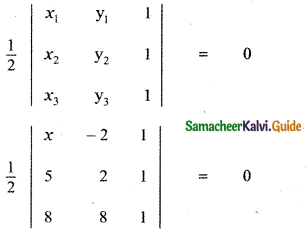$$\frac{1}{2}$$ [x(2 – 8) + 2(5 – 8) + 1(40 – 16)] = 0
x × – 6 + 2 × – 3 + 1 × 24 = 0
– 6x – 6 + 24 = 0
– 6x + 18 = 0
6x = 18 ⇒ x = $$\frac{18}{6}$$ = 3
x = 3

Question 13.
If $$\left| \begin{matrix} 2a & { x }_{ 1 } & { y }_{ 1 } \\ 2b & { x }_{ 2 } & { y }_{ 2 } \\ 2c & { x }_{ 3 } & { y }_{ 3 } \end{matrix} \right|$$ = $$\frac{\text { abc }}{2}$$ ≠ 0, then the area of the triangle whose vertices are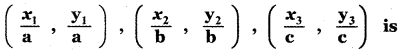(1) $$\frac{1}{4}$$
(2) $$\frac{1}{4}$$abc
(3) $$\frac{1}{8}$$
(4) $$\frac{1}{8}$$abc
(3) $$\frac{1}{8}$$

Explaination: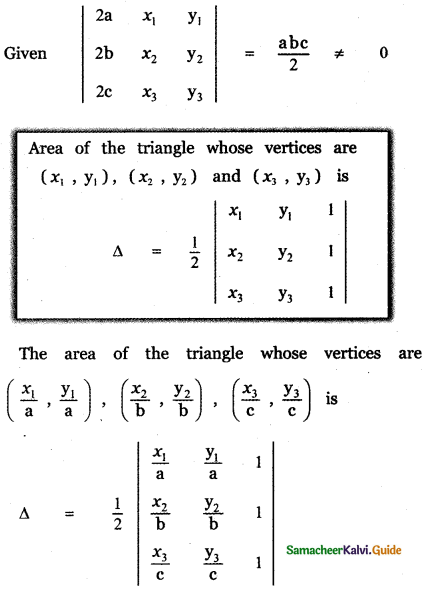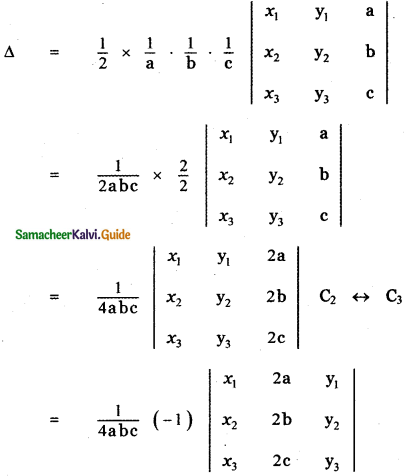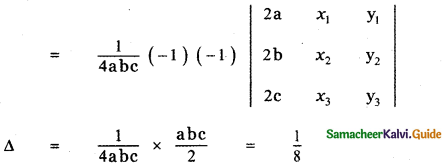Question 14.
If the square of the matrix $$\left[ \begin{matrix} α & β \\ γ & -α \end{matrix} \right]$$ is the unit matrix of order 2, then α, β, and γ should
(1) 1 + α2 + βγ = 0
(2) 1 – α2 – βγ = 0
(3) 1 – α2 + βγ = 0
(4) 1 + α2 – βγ = 0
(1) 1 + α2 + βγ = 0

Explaination:– α2 – βγ = 1
α2 + βγ + 1 = 0

Question 15.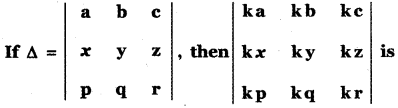(1) Δ
(2) kΔ
(3) 3 kΔ
(4) k3 Δ
(4) k3 Δ

Explaination: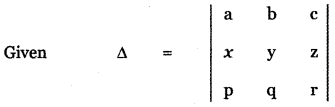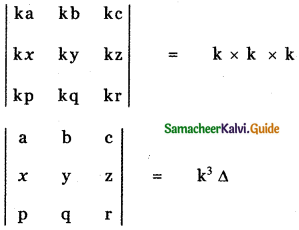Question 16.
A root of the equation $$\left| \begin{matrix} 3-x & -6 & 3 \\ -6 & 3-x & 3 \\ 3 & 3 & -6-x \end{matrix} \right|$$ = 0 is
(1) 6
(2) 3
(3) 0
(4) -6
(3) 0

Explaination: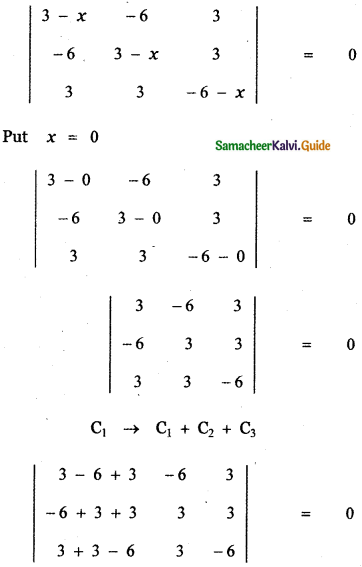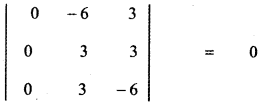0 = 0
x = 0 satisfies the given equation.
Hence the root of the given equation is x = 0.Question 17.
The value of the determinant of A = $$\left[ \begin{matrix} 0 & a & -b \\ -a & 0 & c \\ b & -c & 0 \end{matrix} \right]$$ is
(1) -2 abc
(2) abc
(3) 0
(4) a2 + b2 + c2
(3) 0

Explaination: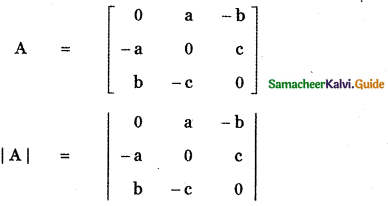= 0 – a(0 – bc) – b (ac – 0)
= abc – abc = 0

Question 18.
If x1, x2, x3 as well as y1, y2, y3 are in geometric progression with the same common ratio, then the points (x1, y1), (x2, y2), (x3, y3) are
(1) vertices of an equilateral triangle
(2) vertices of a right angled triangle
(3) vertices of a right angled isosceles triangle
(4) collinear
(4) collinear

Explaination:
Given x1, x2 , x3, as well as y1, y2, y3, are in geometric progression with the same common ratio.
∴ x1 = a, x2 = ar, x3 = ar2 ,
y1 = b, y2 = br, y3 = br2
(x1, y1) = (a, b),
(x2, y2) = (ar ,br)
and (x3, y3) = (ar2, br2)
Area of the triangle whose vertices are
(x1, y1),(x2, y2) and (x3 y3) is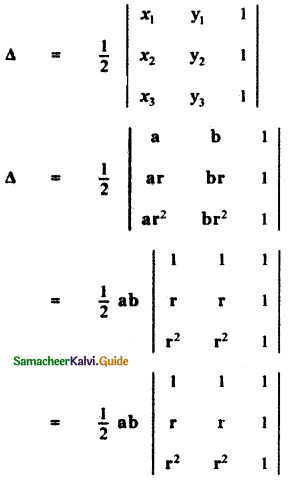= $$\frac{1}{2}$$ab[1(r – r2) – 1 (r – r2) + 1 (r3 – r3)]
= $$\frac{1}{2}$$ab[r – r2 – r + r2 + 0]
= $$\frac{1}{2}$$ab × 0 = 0
∴ The points (x1, y1),(x2, y2) and (x3 y3) are collinear.Question 19.
If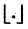denotes the greatest integer less than or equal to the real number under consideration and – 1 ≤ x< 0, 0 ≤ y < 1, 1 ≤ z < 2 , then the value of the determinant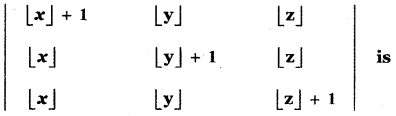(1)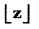(2)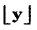(3)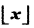(4)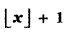(1)Explaination: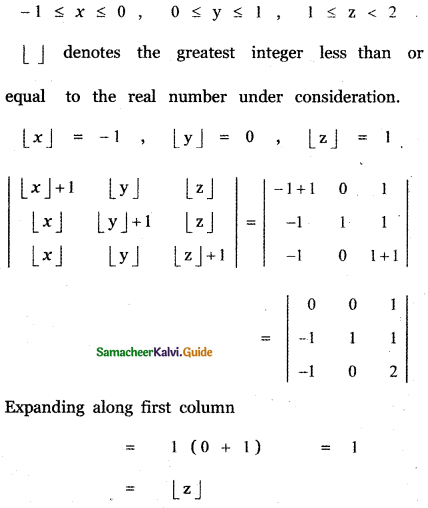Question 20.
If a ≠ b, b, c satisfy $$\left| \begin{matrix} a & 2b & 2c \\ 3 & b & c \\ 4 & a & b \end{matrix} \right|$$ = 0 then abc =
(1) a + b + c
(2) 0
(3) b3
(4) ab + bc
(3) b3

Explaination: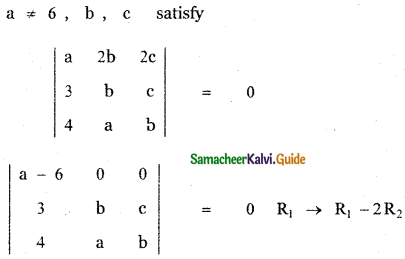(a – 6) (b2 – ac) = 0
Since a ≠ 6 , we have a – 6 ≠ 0
∴ b2 – ac = 0
b2 = ac
b3 = abcQuestion 21.
If A = $$\left| \begin{matrix} -1 & 2 & 4 \\ 3 & 1 & 0 \\ -2 & 4 & 2 \end{matrix} \right|$$ and B = $$\left| \begin{matrix} -2 & 4 & 2 \\ 6 & 2 & 0 \\ -2 & 4 & 8 \end{matrix} \right|$$, then B is given by
(1) B = 4A
(2) B = – 4A
(3) B = – A
(4) B = 6A
(2) B = – 4A

Explaination: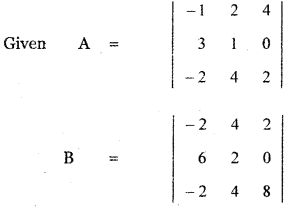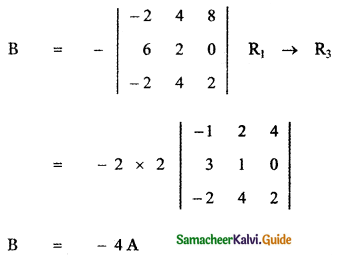Question 22.
IfA skew-symmetric of order n and C is a column matrix of order n × 1, then CT AC is
(1) an identity matrix of order n
(2) an identity matrix of order I
(3) a zero matrix of order 1
(4) an identity matrix of order 2
(3) a zero matrix of order 1

Explaination:
Given A is a skew symmetric matrix of order n.
∴ AT = – A
C is a column matrix of order n × 1
CTis of order 1 × n
∴ CT A is of order (1 × n) × (n × n) = (1 × n)
CTAC is of order (1 × n) × (n × 1) = (1 × 1)
Since A is a skew – symmetric matrix, we have
AT = -A
(CT AC)T= CT AT (CT)T = CT (-A) C
= -CT AC
∴ CTAC is a skew – symmetric matrix.
A matrix of order 1 is skew – symmetric if A is zero matrix.
Since CTAC is a skew – symmetric and its order is 1.
∴ CTAC is a zero matrix.Question 23.
The matrix A satisfying the equation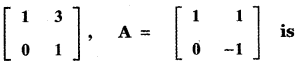(1)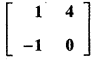(2)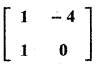(3)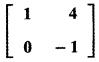(4)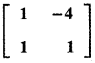(3)Explaination: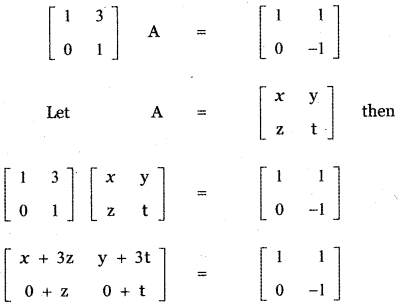x + 3z = 1 ——– (1)
y + 3t = 1 ——- (2)
z = 0
t = – 1
(1) ⇒ x + 3 × 0 = 1 ⇒ x = 1
(2) ⇒ y + 3 × – 1 = 1 ⇒ y = 4
∴ A = $$\left[ \begin{matrix} 1 & 4 \\ 0 & -1 \end{matrix} \right]$$

Question 24.
If A + 1 = $$\left[ \begin{matrix} 3 & -2 \\ 4 & 1 \end{matrix} \right]$$, then (A + I) (A – I) is equal to
(1)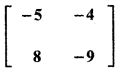(2)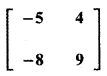(3)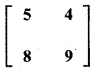(4)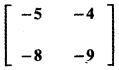(1)Explaination: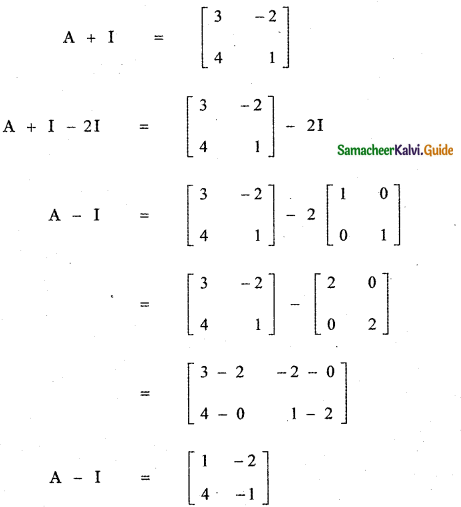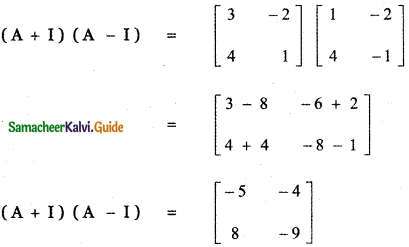Question 25.
Let A and B be two symmetrh matrices of same order. Then which one of the following statement is not true?
(1) A + B is a symmetric matrix
(2) AB is a symmetric matrix
(3) AB = (BA)T
(4) ATB = MIT
(2) AB is a symmetric matrix

Explaination:
Given A and B are two symmetric matrices of the same order.
A = AT , B = BT
(1)(A+B)T = AT + BT = A + B
A + B is symmetric.

(2) (AB)T = BT AT BA
Thus (AB)T ≠ AB
Hence , AB is not symmetric.

(3) AB = (BA)T
= AT BT = AB
Statement is true.

(4) AT B = ABT Since AT = A
B = BT
Statement is true.